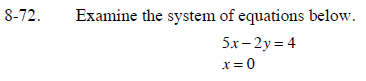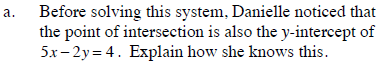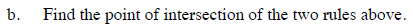### Home > CCA > Chapter 8 > Lesson 8.2.2 > Problem8-72

8-72.
1.Examine the system of equations below. Homework Help ✎

 5x − 2y = 4 x = 0
1. Before solving this system, Danielle noticed that the point of intersection is also the y-intercept of 5x − 2y = 4. Explain how she knows this.

2. Find the point of intersection of the two rules above.The line x = 0 is the y-axis.